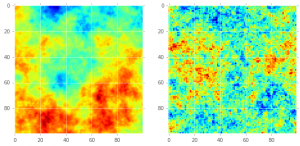# Simulating a spatial Conditional Autoregressive Model (CAR) from a graph G(V,E)

This is the second delivery on how to simulate a Conditional Autoregressive Model (CAR) using a given graph (aka, lattice, network, etc). For simulating a CAR model without a given graph see: [ HERE ].

## Ingredients

• An adjacency matrix (M) representation of a lattice. This represented a simple graph, i.e. ($$\delta_{i,j} = 1$$) if there exists an edge between $$i \text{and} j$$.
• [To Plot] A corresponding data frame (GeoPandas) to visualize polygons corresponding to the areas of the lattice.

## Methods

Load the necessary data. In this example, the lattice L corresponds is the following figure

## Geometry file
geometryfile = "training_data_sample_puebla_p9_geometry.json"
gg.set_index('indx',inplace=True)
plt.rcParams['figure.figsize'] = [10, 10]
gg.plot(edgecolor='black')
M = np.load(file=mat_filename)

The order of the columns (rows) of M corresponds to the ascending order of the indices of the polygons in the GeoPandas Data Frame, i.e. the element in gg with the lowest index corresponds to the first column (row) of M, and successively. If this order is not respected the spatial autocorrelation will be lost.

## Building the precision matrix Q

Given that the probability distribution of CAR model is a Multivariate Normal distribution $$MVN(0,Q^{-1})$$. Simulating a CAR model would correspond to simulating this process. The challenge resides, however, in the construction of the precision matrix $$Q$$. We will follow the work of: Besag, 1991 , Rue and Hoavard, 2005 and Morris et. al. 2019 . Any doubt go those references.

During the realisation of this tutorial I had problems simulating an intrinsic CAR Model obtaining a Q that is not semi positive definite (SPD). In this regard we will build Q as follows:

$$Q = \tau^2 D_{w}( I – \alpha B)$$

Where $$\tau^2$$ models the general variance, $$D_{w}$$ represents the number of neighbours for each node in the graph and B is the matrix resulting from $$B = M/D_{w}$$ where M is the corresponding adjacency matrix. B guarantees that Q will be positive definite. The parameter $$\alpha$$ represents the degree of spatial autocorrelation and ranges from $$(0 to 1)$$. I recommend to test different values of $$\alpha$$. In addition remove any “islands” nodes without neighbours. Failure to do so can lead to no positive definiteness. The code to achieve this is here:

def buildQ(M,D,tau_2=2.0,alpha=0.99999):
I = np.identity(M.shape)
D_ = np.diag(np.reciprocal(np.diag(D)))
B = np.dot(M,D_)
Q = np.dot(tau_2 * D_,(I - alpha* B))
return(Q)

## Calculate number of neighbours
D = np.sum(M,axis=1)
## Remove islands
idx_islands = np.where(D == 0 )
ND = np.delete(D,idx_islands,axis=0)
NM = np.delete(M,idx_islands,axis=0)
NM = np.delete(NM,idx_islands,axis=1)

## Calculate Q
Q = buildQ(NM,ND,tau_2=2.0,alpha=0.995)

Here we will visualize the Covariance matrix ($$Q^{-1}$$)

A we now made sure (by construction, or try and error 😛 ) that Q is Positive Definite, we can therefore calculate the Cholesky decomposition, making the simulation remarkably fast and efficient. Here we follow algorithm 2.4 from Rue and Hovard. The code is as follows:

## Simulation Algorithm 2.4
def generateCARSimulation(Q,mu):
L = np.linalg.cholesky(Q)
I = np.identity(L.shape)
z = sp.random.normal(loc=mu,scale=1.0,size=L.shape)
v = np.linalg.solve(L.transpose(),z)
return(v)v = generateCARSimulation(Q,mu)

To visualise the simulation just join the random vector v with the GeoPandas dataframe, gg as follows.

## order index (cell_id) ascending, according to M.
ordered_g = gg.sort_index()
## Reset the index, this is necessary in order to join.
ordered_g.reset_index(inplace=True)
## Create the dataframe
yy = pd.DataFrame(vs,columns=['simulated'])
## Join it with the geospatial dataframe
simulation = ordered_g.join(yy)
## Plot it!
simulation.plot(column='simulated')

This is the visualisation.

Thanks for reading and remember to use your skills for the benefit of Mother Earth.

# Stationary GMRF simulation with Discrete Fourier Transformation

### Algorithm to simulate GMRF with block-circulant Matrix.

Algorithm 2.10

Taken from: Rue, H., & Held, L. (2005). Gaussian Markov random fields: theory and applications. CRC press.

### let’s build the circulant matrix for the tourus

Oke, for the moment I´ll follow the example in GMRF book. i.e. a Torus (stationary of 128×128)

def createToroidalCircularBase(d=0.1,N=128):
"""
Creates a circular base similar to the one described in GMRF Rue and Held, 2005.
"""
c00 = np.hstack(((4 + d),-1,np.zeros(N - 3),-1))
c01 = np.hstack((-1,np.zeros(N - 1)))
c0 = np.zeros((N - 2 ,N))
c1 = np.vstack((c00,c01))
c = np.vstack((c1,c0))
c[N -1, 0] = -1
return c



%%time
## Create circular base
d = 0.00001
N = 100
c = createToroidalCircularBase(d=d,N=N)
## Simulate random noise (Normal distributed)
from scipy.fftpack import ifft2, fft2
zr = scipy.stats.norm.rvs(size=(c.size,2),loc=0,scale=1,random_state=1234)
zr.dtype=np.complex_
#plt.hist(zr.real)
#Lm = scipy.sqrt(C.shape*C.shape) * fft2(C)

Lm = fft2(c)
v = 1.0/ len(c) * fft2((Lm ** -0.5)* zr.reshape(Lm.shape))
x = v.real
plt.imshow(x,interpolation='None')
Both figures represent a simulation on a 100×100 cells. The comparison is as follows: on the right there is full gaussian field with mean zero and some Matern-based covariance structure. On the right a Markov Random Field simulation using the method described above.## [Bonus] Create a simple precision matrix

An easy way to create a precision matrix is with the function: *diags* inside the module: scipy.sparse. The code will look like this:

from scipy.sparse import diags
N = 10
diagonals = [np.zeros(N), np.ones(N-1), np.ones(N-1)]
# order 0 := diagonal , 1 first on top of diagonal, -1 first bottom of diagonal
offset = [0,1,-1]
## maps diagonals with position (offset)
M = diags(diagonals,offset).toarray()
## "seal the edges"
M = 1
M[N-1][N-1] =1
print(M)

Result:

[[ 1.  1.  0.  0.  0.  0.  0.  0.  0.  0.]
[ 1.  0.  1.  0.  0.  0.  0.  0.  0.  0.]
[ 0.  1.  0.  1.  0.  0.  0.  0.  0.  0.]
[ 0.  0.  1.  0.  1.  0.  0.  0.  0.  0.]
[ 0.  0.  0.  1.  0.  1.  0.  0.  0.  0.]
[ 0.  0.  0.  0.  1.  0.  1.  0.  0.  0.]
[ 0.  0.  0.  0.  0.  1.  0.  1.  0.  0.]
[ 0.  0.  0.  0.  0.  0.  1.  0.  1.  0.]
[ 0.  0.  0.  0.  0.  0.  0.  1.  0.  1.]
[ 0.  0.  0.  0.  0.  0.  0.  0.  1.  1.]]

## Update

There is a new tutorial for simulating a CAR model using a given adjacency matrix and spatial lattice. Go here to read it.